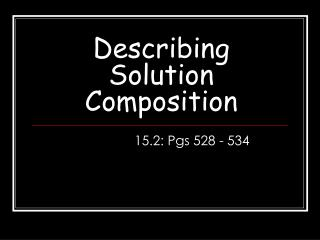# Describing Solution Composition - PowerPoint PPT PresentationDownload PresentationDescribing Solution Composition

Describing Solution CompositionDownload Presentation## Describing Solution Composition

- - - - - - - - - - - - - - - - - - - - - - - - - - - E N D - - - - - - - - - - - - - - - - - - - - - - - - - - -
##### Presentation Transcript

1. Describing Solution Composition 15.2: Pgs 528 - 534

2. Mass Percent • Describes the amount of solution in terms of mass • Suppose a solution is made by dissolving 13.4g of NaCl in 56.6 mL of water. What is the mass percent of NaCl in this solution?

3. Solution Concentration • Molarity: number of moles of solute per liters of solution • Molarity 

4. Solution Concentration • Molarity – What is the molarity of a 1 L solution containing 6 moles of sugar? What is the molarity if the 6 moles of sugar is dissolved in only 500 mL of solution?

5. Example: • What is the molarity if 11.5 g of NaOH is added to 1.5 L of water?

6. Practice!! • What is the molarity if 1.56 g of HCl is added to 26.8 mL of solution.

7. Practice 2!! • How many grams of formaldehyde (HCHO) must be used to prepare 2.5 L of 12.3 M solution?

8. Solution Concentration • Standard Solution – when the concentration is accurately known. • Made using a volumetric flask